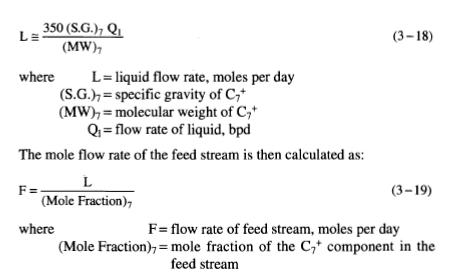﻿ The Flow Stream | Oil & Gas Process Engineering

## The Flow Stream

Many times the designer is given the mole fraction of each component in the feed stream but is not given the mole flow rate for the stream. It may be necessary to estimate the total number of moles in the feed stream (F) from an expected stock tank oil flow rate. As a first approximation, it can be assumed that all the oil in the stock tank can be characterized by the C7+ component of the stream. Thus, the feed rate in moles per day can be approximated as:For our example, if the mole feed rate of 10,000 moles per day was not given, but was required to design for 2,500 bpd of stock tank liquid, the mole feed rate for flash calculations would be approximated as:The flash calculation could then proceed. The calculated flow rates for each stream in the process could then be ratioed to reflect the error between assumed stock tank flow rate and desired stock tank flow rate.